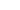2010 scott voltage fr

• + 1LoganSTP (Aug 17, 2009 at 23:17)
˙ɹıɐ uɐ ɥʇıʍ ɹǝןooɔ ʎɐʍ sʞooן ʇı ˙ʎןƃnɟ ƃuıʞooן ʎq ʇı sןıods ʇı
• + 1Leftwing29 (Aug 19, 2009 at 17:42)
eh, i like the coil shock better.
• + 2Gitan (Jul 13, 2009 at 13:13)
yess sexy !
• + 2va7kpt (Jul 21, 2009 at 6:56)
That is a babe of a bike
• + 1LoganSTP (Aug 19, 2009 at 21:11)
ʞuıɥʇ ı ǝɔuǝɹǝɟǝɹd ןɐuosɹǝd
• + 1BuckyWeston (Aug 22, 2009 at 10:23)
i kinda agree with you you on that one
• + 1joram-adams (Sep 1, 2009 at 23:31)
Luv it! how do you do that upside down writing?
• + 1LoganSTP (Sep 1, 2009 at 23:44)
• + 1marlon-d (Sep 28, 2009 at 11:51)
what model fr is it??? its the cooolest
• + 1studers (Jun 9, 2010 at 12:49)
howd u get a different paint job? and how much?
• + 1LoganSTP (Jun 10, 2010 at 19:42)
its not mine its a demo one they released before they where produced
• + 1elfreeridefreako (Jul 12, 2009 at 16:06)
sex
• + 1marlon-d (Sep 12, 2009 at 18:51)
ual! i want
• + 1LoganSTP (Jul 12, 2009 at 17:44)
ohh yes
• + 1amplid (Aug 3, 2009 at 13:32)
sick je• + 1LoganSTP (Sep 29, 2009 at 3:14)
its just a custom one.
• + 1studers (Jun 13, 2010 at 10:18)
oh cool
• - 3LoganSTP (Jul 21, 2009 at 23:55)
˙ʇı sןıods ɐpuıʞ ʞɔoɥs ןıoɔ ǝɥʇ ʇnq ɥɐǝʎ
• + 1Leftwing29 (Aug 17, 2009 at 21:48)
how does the coil shock spoil it?